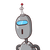# Determine whether they are in proportion. 21,49,33,77 Give full explanation

Determine whether they are in proportion.
21,49,33,77
Give full explanation

### 1 thought on “Determine whether they are in proportion.<br /> 21,49,33,77<br /> Give full explanation”

1.$$\frac{21}{49} = \frac{33}{77} \\ \\ \frac{3}{7} = \frac{3}{7} \: \: so \: the \: fractions \: are \: equal \: now \: finding \: the \: mutipe \: of \: iterior \: and \: exterior$$